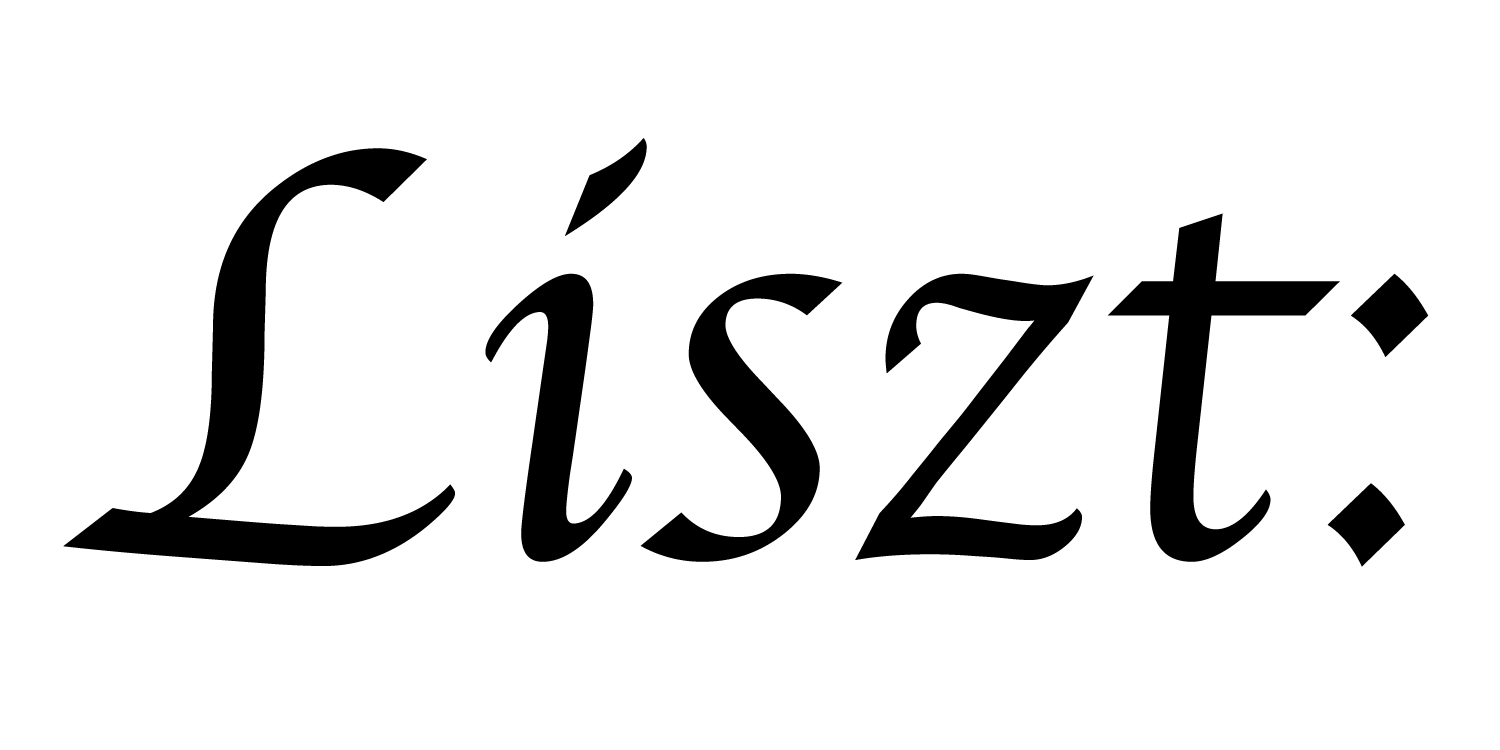# 10: Data Layout Descriptors (DLDs)

In this example, we’ll see how to get lower level access to the data that Ebb is storing and computing on. This data access is provided through metadata objects that we call data layout descriptors (DLDs). These descriptors communicate information about how data is stored in memory so that Ebb-external code can directly manipulate the data without incurring memory copy overheads.

``````import 'ebb'
local L = require 'ebblib'

local GridLib   = require 'ebb.domains.grid'

local DLD       = require 'ebb.lib.dld'
local vdb       = require 'ebb.lib.vdb'
``````

In addition to libraries we’ve already seen, we also require the DLD library.

``````local N = 40

local grid = GridLib.NewGrid2d {
size   = {N, N},
origin = {0, 0},
width  = {N, N},
periodic_boundary = {true,true},
}

local timestep    = L.Constant(L.double, 0.45)
local conduction  = L.Constant(L.double, 1.0)

local function init_temperature(x_idx, y_idx)
if x_idx == 4 and y_idx == 6 then return 400
else return 0 end
end

local ebb visualize ( c : grid.cells )
vdb.color({ 0.5 * c.t + 0.5, 0.5-c.t, 0.5-c.t })
var p2 = c.center
vdb.point({ p2, p2, 0 })
end

local ebb update_temperature ( c : grid.cells )
var avg   = (1.0/4.0) * ( c(1,0).t + c(-1,0).t + c(0,1).t + c(0,-1).t )
var diff  = avg - c.t
c.new_t   = c.t + timestep * conduction * diff
end
``````

Similarly to tutorial 09, we simplify the grid-based heat-diffusion code by assuming periodic boundaries.

Below we define a function to shuffle the temperature data in a way that would not be allowed by an Ebb `foreach()` call. This function is defined using Terra, which is a C-alternative language embedded in Lua. When using Ebb, Terra is always available to you. We could equally well use a function defined in C, and do exactly that in a later tutorial.

Using DLDs, we can incorporate pre-existing linear solvers, an FFT, or other computations that are (i) legacy code, (ii) specially optimized, or (iii) use computation patterns that Ebb does not support or allow.

``````terra tile_shuffle( dld : &DLD.C_DLD )
var xstride = dld.dim_stride
var ystride = dld.dim_stride

for y=0,20 do
for x=0,20 do
var t1_idx  =      x * xstride   +      y * ystride
var t2_idx  = (x+20) * xstride   + (y+20) * ystride

var temp      = t_ptr[t1_idx]
t_ptr[t1_idx] = t_ptr[t2_idx]
t_ptr[t2_idx] = temp
end
end
end
``````

This Terra function reads out the address of the temperature data and the appropriate strides to let it iterate over that data. Because we’re writing this function for the very specific case of a 40x40 2d grid of double values, we can simplify the code tremendously. The function swaps the top left quadrant with the bottom right. This function doesn’t have any physical analogy, but it’s a simple example of a computation that we can’t encode in Ebb due to phase-checking restrictions.

While using these external computations imposes less restrictions, we also lose Ebb’s ability to automatically parallelize the code. The `tile_shuffle` function depends on data being CPU-resident, and computes the swap as a purely sequential computation. If we want to swap in parallel on a GPU, then we would have to write an entirely separate function.

``````for i=1,360 do
grid.cells:foreach(update_temperature)
grid.cells:Swap('t', 'new_t')

if i % 200 == 199 then
local t_dld = grid.cells.t:GetDLD()

assert( t_dld.version == 1 and t_dld.version == 0 )
assert( t_dld.base_type      == DLD.DOUBLE )
assert( t_dld.location       == DLD.CPU )
assert( t_dld.type_stride    == 8 )
assert( t_dld.type_dims == 1 and t_dld.type_dims == 1 )
assert( t_dld.dim_size == 40 and
t_dld.dim_size == 40 and
t_dld.dim_size == 1 )

tile_shuffle( t_dld:toTerra() )
end

vdb.vbegin()
vdb.frame()
grid.cells:foreach(visualize)
vdb.vend()

if i % 10 == 0 then print( 'iteration #'..tostring(i) ) end
end
``````

Finally, we modify our simulation loop to swap the tiles on the 200th iteration. This swap proceeds by requesting a Lua form of the DLD object via `:GetDLD()`, asserting that a number of values are what we expect them to be, and finally calling the tile_shuffle function with a Terra version of the DLD object.

a part of the Liszt project and PSAAP II center at Stanford University